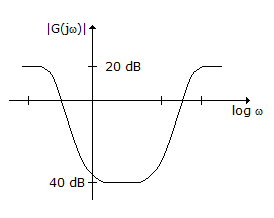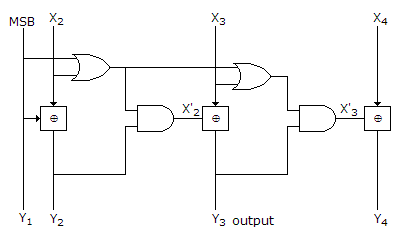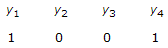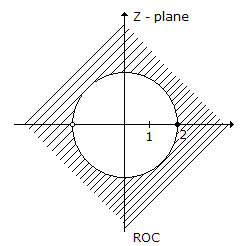# Electronics and Communication Engineering - Exam Questions Papers

41.

The magnitude plot of a rational transfer function G(s) with real coefficients is shown below. Which of the following compensators has such a magnitude plot?A. Lead compensator B. Lag compensator C. PID compensator D. Lead-lag compensator

Explanation:

Since magnitude plot shows both increasing as well as decreasing plot, it is lead-lag compensator.

42.

The circuit shown in the figure convertsA. BCD to Binary code B. Binary to excess - 3 code C. Excess - 3 to Gray D. Gray to Binary

Explanation:

Let input is 1 1 0 1

y1 = x1y2 = x2x1 ⇒ 1 ⊕ 1 = 0

y3 = x3x2 = 0 ⊕ 0 = 0

y4 = x4x3 = 1 ⊕ 0 = 1

if input is gray then y1 y2 y3 y4 will be Binary.

43.

Using 4-bit numbers (n = 4) if k = (0011)2 how is k expressed in 2's complement.

 A. (1011)2 B. (1101)2 C. (1100)2 D. (0101)2

Explanation:

In 2's complement

Simply find 2s complement

⇒ (0011)2 ⇒ 1100 + 1 ⇒ (1101)2 .

44.

Which of the following options is the closest in meaning to the world below:
Circuitous

 A. cyclic B. indirect C. confusing D. crooked

Explanation:

Circuitous means round about or not direct. Therefore the closest in meaning will be indirect.

45.

System is :A. causal B. stable C. non causal D. anticausal